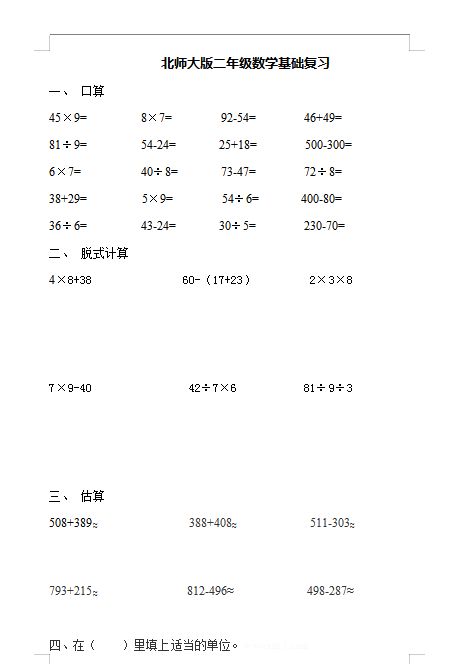• 口算

45×9=         8×7=        92-54=        46+49=

81÷9=         54-24=       25+18=        500-300=

6×7=          40÷8=       73-47=        72÷8=

38+29=         5×9=        54÷6=       400-80=

36÷6=         43-24=       30÷5=        230-70=

• 脱式计算

4×8+38               60-（17+23）         2×3×8

2022：新北师大版小学数学2年级下册基础知识 (2)（doc文档4电页子档下载）